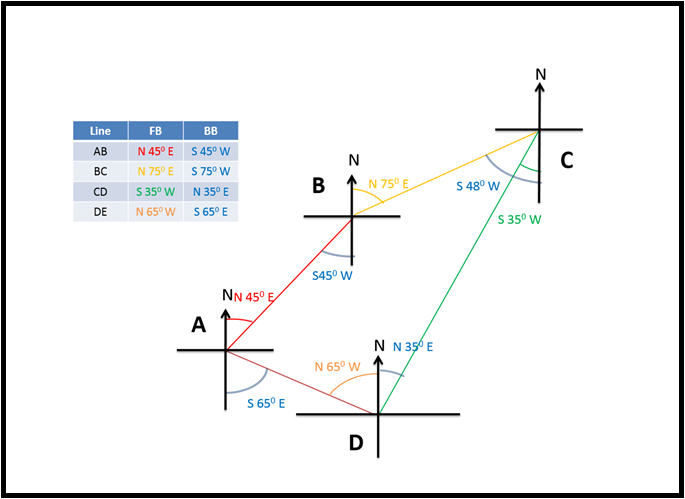# Basic Civil Engineering (3110004)

BE | Semester-1   Winter-2019 | 08-01-2020

## Q5) (c)

#### Given below are the bearings of lines of a closed traverse ABCD. Calculate the interior angles. Line F.B. AB N45°E BC N75°E CD S35°W DA N65°W

∠A = BB of line EA – FB of line AB = 180° – 45° – 65° = 70°

∠B = BB of line AB – FB of line BC = 180° – 75° + 45° = 150°

∠C = 360° – FB of line CD + BB of line BC = 75° – 35° = 40°

∠D= 360° – FB of line DE + BB of line CD = 65° + 35° = 100°

Sum of Included Angles = ∠A + ∠B + ∠C + ∠D = 70° + 150° + 40° + 100° = 360°

Check = (2N - 4) X 90° = 360°

Here sum of included angles = check   (hence O.K.)